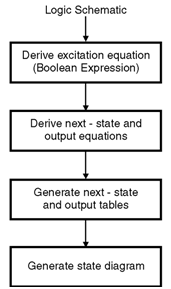Home > finite state machines > State Machine Fundamentals > Analysis of Sequential Circuits

# Analysis-of-Sequential-Circuits

Analysis of Sequential Circuits : The behaviour of a sequential circuit is determined from the inputs, the outputs and the states of its flip-flops. Both the output and the next state are a function of the inputs and the present state. The analysis task is much simpler than the synthesis task. To analyze a circuit, we simply reverse the steps of synthesis process. Figure below shows the analysis steps.Analysis procedure of a sequential circuit :

1. We start with the logic schematic from which we can derive excitation equations for each flip-flop input.
2. Then, to obtain next-state equations, we insert the excitation equations into the characteristic equations.
3. The output equations can be derived from the schematic, and once we have our output and next-state equations, we can generate the next-state and output tables as well as state diagrams.
4. When we reach this stage, we use either the table or the state diagram to develop a timing diagram which can be verified through simulation.